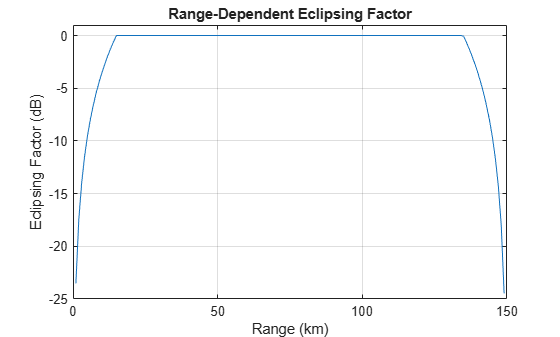eclipsingfactor

Range-dependent eclipsing factor

Description

example

FECL = eclipsingfactor(R,DU,PRF) computes the range-dependent eclipsing factor FECL in decibels, given unambiguous range R duty cycle for a simple rectangular pulse or vector of samples from an arbitrary waveform DU and pulse repetition frequency PRF.

Examples

collapse all

Calculate the range-dependent eclipsing factor at 1 km intervals between zero and the unambiguous range, R, assuming an unmodulated rectangular pulse with a duty cycle of 0.1 and the pulse repetition frequency of 1000 Hz.

DU = 0.1;
PRF = 1e3;
R = 0:1000:time2range(1/PRF);
FECL = eclipsingfactor(R,DU,PRF);

Plot the range-dependent eclipsing factor.

plot(R*1e-3,FECL)
xlabel('Range (km)');
ylabel('Eclipsing Factor (dB)');
ylim([-25 1]);
grid on;
title('Range-Dependent Eclipsing Factor');Input Arguments

collapse all

Range at which to compute the eclipsing factor, specified as a positive scalar or as a length-J vector in meters.

Duty cycle, specified as a nonnegative scalar in the range [0,1] or length-M vector with each element in the range [0,1].

• If you specify DU as a scalar, the eclipsing factor is computed for an unmodulated rectangular pulse with the specified duty cycle.

• If you specify DU as a length-M vector, the eclipsing factor is computed for a waveform, using time domain samples taken over a one-pulse interval.

Pulse repetition frequency, specified as a positive scalar or as a length-K vector in Hz.

Output Arguments

collapse all

Eclipsing factor, returned as a J-by-K matrix in decibels with rows corresponding to the ranges in R and columns corresponding to the values in PRF.

Extended Capabilities

C/C++ Code GenerationGenerate C and C++ code using MATLAB® Coder™.